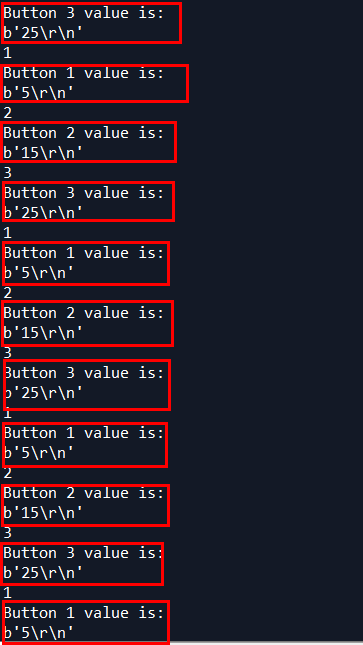Intefacing week - Group assignment

Using Python to read the Arduino's output via Serial! For this, the Python package pySerial needs to be installed.
Following the simple documentation:
https://pythonhosted.org/pyserial/shortintro.htmlA python programmer lol

Programming the Arduino with the code:

#include <SoftwareSerial.h> #define rxPin 0 #define txPin 1 int val = 0; SoftwareSerial my_serial(rxPin, txPin); void setup() { pinMode(rxPin,INPUT); pinMode(txPin,OUTPUT); my_serial.begin(9600); } void loop() { val = 5; my_serial.println(val); val = 15; my_serial.println(val); val = 25; my_serial.println(val); delay(10); }

In Python, we imported the module and declared the serial:

import serial my_serial = serial.Serial('COM4', 9600)

The Arduino, as shown in the code, does the following:
Every loop cycle, it prints out three values - 5, 15 and 25 (arbitrarily chosen). With Python - we will read these three values, in a row, and assign each one to it's own variable. This is for the sake of testing how to get multiple values from multiple inputs (such as several different buttons) and to assign them correctly and make the data usable.
To get the three different values in python each time and assign to each one it's own variable, we used a simple counter logic:

counter = 0 #we'll be iterating 3 variables in the loop each time # value 1, 2 and 3 are the variables to assign the values read from the serial port to value1=0 value2=0 value3=0 # running this loop - from what Emma explained, if in the Arduino there is one loop cycle that has three different values sent through serial # in the actual code - looping through the input three times is how to get all three values from the single Arduino cycle # in short - **Three** cycles in the python code to read 3 values generated within **One** cycle of the Arduino code while True: counter = counter + 1 print(counter) if counter == 1: value1 = my_serial.readline() print("Button 1 value is:") print(value1) elif counter ==2 : value2 = my_serial.readline() print("Button 2 value is:") print(value2) elif counter == 3: value3 = my_serial.readline() print("Button 3 value is:") print(value3) counter = 0

The code basically means that:
As the Arduino writes to the serial 5, 15 and 25 respectively - in the python reading of the serial, 5 will always be assigned to the variable "value1", 15 always be assigned to the variable "value2", and 25 always assigned to "value3".
The results of course work:The values aren't trimmed for unnecessary additional characters, so we get the string "5\r\n" instead of just 5, but it's consistent and serves the current purpose of this demonstration. The additional printed numbers seen in the screenshot, 1, 2 and 3, are just the result of the "print(counter)" command which is only for debugging purposes.

Full Python code for reference:

import serial my_serial = serial.Serial('COM4', 9600) print(my_serial.name) counter = 0 value1=0 value2=0 value3=0 while True: #print(counter) counter = counter + 1 #print(counter) if counter == 1: value1 = my_serial.readline() print("Button 1 value is:") print(value1) elif counter ==2 : value2 = my_serial.readline() print("Button 2 value is:") print(value2) elif counter == 3: value3 = my_serial.readline() print("Button 3 value is:") print(value3) counter = 0

The .py code file: